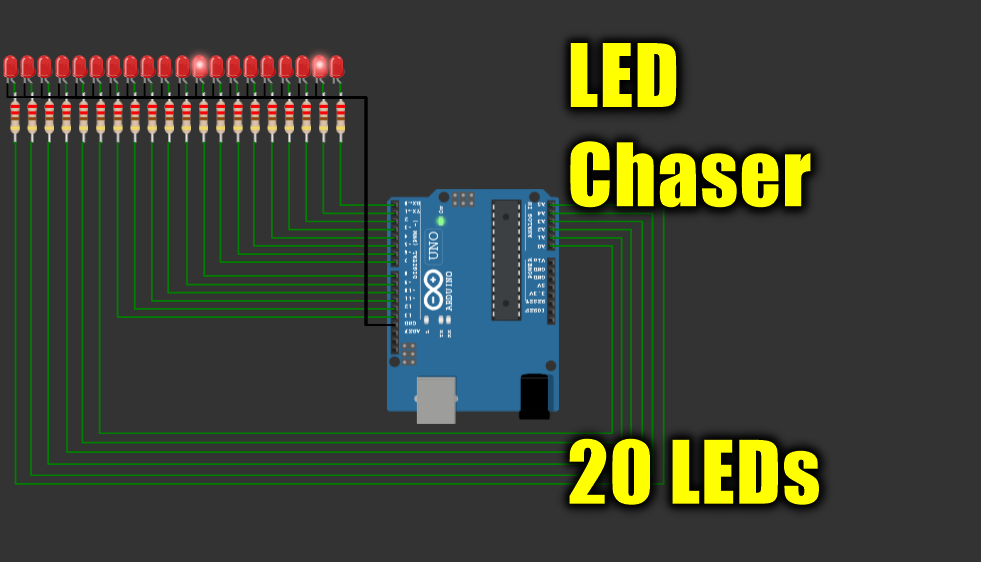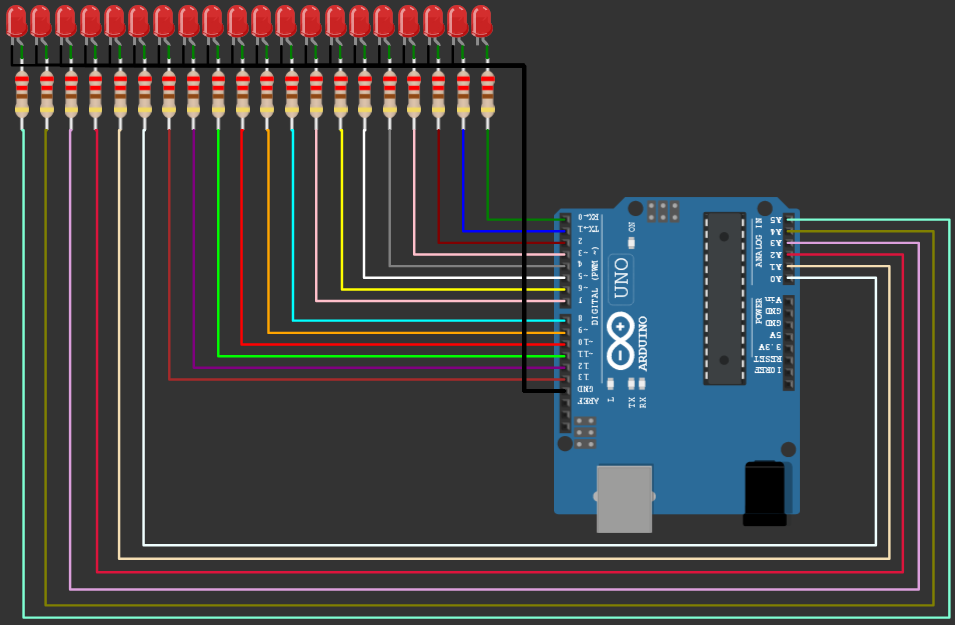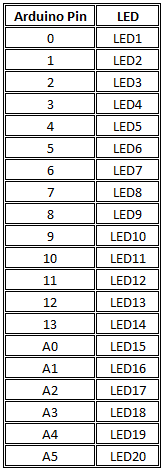Arduino

# Online Simulator - 20 LEDs based LED chaser project using Arduino UNOJuly 08, 2021 by share Open-Tech
ShareLED chaser project to create immersing patterns using Arduino UNO and 20 LEDs!

## Hardware

 1 Arduino UNO

In this article, you will find all the necessary information for your next LED chaser project. This project can be easily edited by anyone online! I hope you will be able to create more stunning LED projects and have fun. Wokwi Arduino simulator enables to create such projects for free online. Let us get started.

## Connection Diagram

Here is a brief connection diagram showing the connections and the arrangement.LED chaser project 20 LEDs on Wokwi Arduino Simulator

https://wokwi.com/arduino/projects/303372512437207617

## Project brief

you will need: 20 LEDs, connecting wires, a bread board and Arduino UNO. The resistors are used to limit the current through the LEDs which may damage the UNO if missed. I have used 22 ohms resistor but you can also use more or less similar value. We are using 20 GPIO pins of Arduino.

Here is a simple table of pin assignments done in the LED chaser project.LED chaser Pin mapping

## Code for LED chaser

``````void setup()
{
for (int pin = 0; pin <= 19; pin++)
{
pinMode(pin, OUTPUT);
}
}
//Main Loop - Switches different LED Patterns
void loop()
{
for (int j = 0; j < 10 ; j++) {
// onrun(20);
//offrun(50);
//flash(200);
//alternate(200);
//stack(20);
//drawstack(random(1,20));
chaser(50);
}
}
void clearall()
{
for (int pin = 0; pin <= 19; pin++)
{
digitalWrite(pin, LOW);
}
}
void fillall()
{
for (int pin = 0; pin <= 19; pin++)
{
digitalWrite(pin, HIGH);
}
}
//One ON LED Run and all other OFF
void onrun(int delaytime)
{
for (int pin = 0; pin <= 19; pin++)
{
clearall();
digitalWrite(pin, HIGH);
delay(delaytime);
}
for (int pin = 19; pin >= 0; pin--)
{
clearall();
digitalWrite(pin, HIGH);
delay(delaytime);
}
}
//One OFF LED Run and all other OFF
void offrun(int delaytime)
{
for (int pin = 0; pin <= 19; pin++)
{
fillall();
digitalWrite(pin, LOW);
delay(delaytime);
}
for (int pin = 19; pin >= 0; pin--)
{
fillall();
digitalWrite(pin, LOW);
delay(delaytime);
}
}
//Flashing all LEDs ON and OFF
void flash(int delaytime)
{
for (int i = 0; i <= 19; i++)
{
clearall();
delay(delaytime);
fillall();
delay(delaytime);
}
}
//Alternate Flash - Similar to Flash but alternate LEDs
void alternate(int delaytime)
{
for (int n = 1; n <= 5; n++)
{
clearall();
for (int i = 0; i <= 19; i += 2)
{
digitalWrite(i, HIGH);
}
delay(delaytime);
clearall();
for (int j = 1; j <= 19; j += 2)
{
digitalWrite(j, HIGH);
}
delay(delaytime);
}
}
//Putting all LEDs one by one in a stack
void stack(int delaytime)
{
int stack = 0;
while (stack < 20)
{
for (int pos = 0; pos <= (19 - stack); pos++)
{
clearall();
digitalWrite(pos, HIGH);
drawstack(stack);
delay(delaytime);
}
stack++;
}
}
//Subfunction of the stack function
void drawstack(int stack)
{
for (int n = 19; n > (19 - stack); n--)
{
if (n >= 0)
{
digitalWrite(n, HIGH);
delay(20);
}
}
clearall();
}
//One LED chases another LED front and back
void chaser(int delaytime)
{
int div = 40;
int flashtime = delaytime / div;
int A = random(2, 7);
int B = random(7, 12);
int Av = 1;
int Bv = 1;
if (random(0, 2))
{
Av *= -1;
}
if (random(0, 2))
{
Bv *= -1;
}
for (int time = 1; time < 100; time++)
{
if (abs(A - B) == 1 && (Av * Bv) == -1)
{
for (int f = 1; f < round(div / 4); f++)
{
clearall();
delay(flashtime);
digitalWrite(A, HIGH);
digitalWrite(B, HIGH);
delay(flashtime);
}
Av *= -1;
Bv *= -1;
A += Av;
B += Bv;
}
else
{
clearall();
digitalWrite(A, HIGH);
digitalWrite(B, HIGH);
A += Av;
B += Bv;
delay(delaytime);
}
if (A < 0)
{
A = 1;
Av *= -1;
}
if (B > 19)
{
B = 18;
Bv *= -1;
}
if (A >= B)
{
A = B - 1;
}
}
}``````

## Support

For any support or help please visit https://wokwi.com/discord